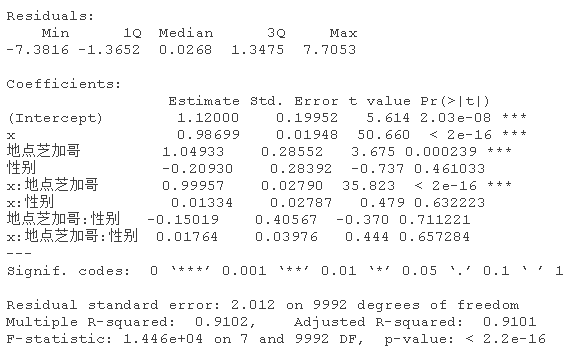# R语言计量经济学：虚拟变量(哑变量)在线性回归模型中的应用

## 例子：性别

``````#真斜率，男性=5，女性=1
ifelse(d\$性别==1, 10+5*d\$x+e,5+d\$x+e)``````

``plot(data=d)``

x的估计系数不正确。

# R语言基于线性回归的资本资产定价模型（CAPM）

## 性别和地点的虚拟变量

### 性别并不重要，但地点很重要

``plot(d,grid~location)``

R-squared是相当低的。

# 正如预期，性别的影响并不显著。### 性别并不重要，而地点会改变截距和斜率

``````ifelse(d\$性别=="0" & d\$地点=="多伦多", 1+1*d\$x+e,
+                    ifelse(d\$性别=="1" & d\$地点=="芝加哥", 20+2*d\$x+e,
+                           ifelse(d\$性别=="0" & d\$地点=="芝加哥", 2+2*d\$x+e,NA))))``````

# 现在让我们获取一些性别和地点都很重要的数据。让我们从两个地点开始。

``plot(d,x,y,color=性别~地点)``

### 性别和地点都很重要，5个地点

``````+                    ifelse(d\$性别=="1" & d\$地点=="芝加哥", 2+10*d\$x+e,
+                           ifelse(d\$性别=="0" & d\$地点=="芝加哥", 2+2*d\$x+e,
+                                  ifelse(d\$性别=="1" & d\$地点=="纽约",3+15*d\$x+e,
+                                         ifelse(d\$性别=="0" & d\$地点=="纽约",3+5*d\$x+e,
+                                                ifelse(d\$性别=="1" & d\$地点=="北京",8+30*d\$x+e,
+                                                       ifelse(d\$性别=="0" & d\$地点=="北京",8+2*d\$x+e,
+                                                              ifelse(d\$性别=="1" & d\$地点=="上海",``````
``plot( x,y,color=性别 ~地点)``

Kaizong Ye拓端研究室（TRL）的研究员。

​非常感谢您阅读本文，如需帮助请联系我们！QQ在线咨询

15121130882

0571-63341498

## 关注有关新文章的微信公众号

This will close in 0 seconds